OpenWindows User's Guide

# Modes of Operation

The Calculator has various modes of operation. The area below the window header displays the current number, followed by a mode line that displays the current mode settings.

## Numeric Bases

You can set the numeric bases to Binary, Octal, Decimal, or Hexadecimal using the Base key pop-up menu, as shown in Figure 8-2. Press MENU on the Base key to display the menu.

##### Figure 8-2 Numeric Base Pop-up Menu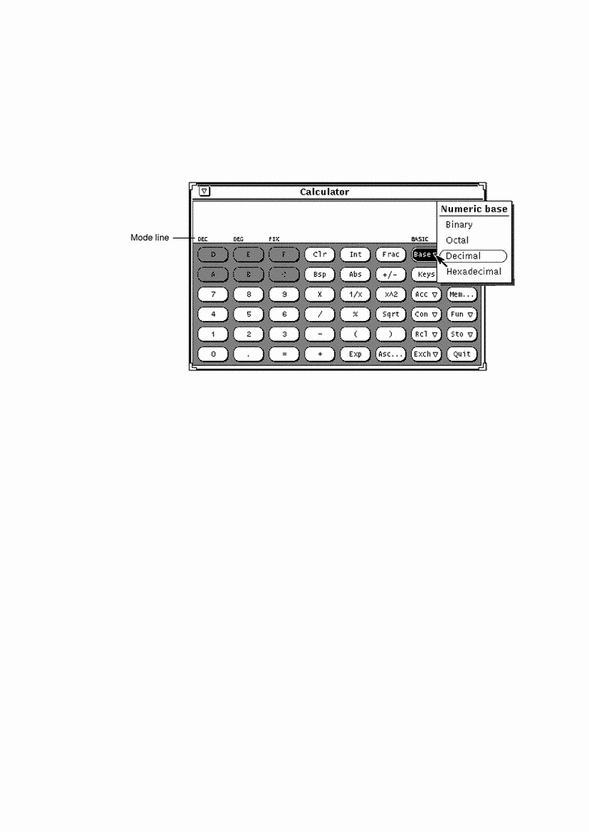The current base setting is shown as the first item in the mode line. In Figure 8-2, "DEC" indicates Decimal base.

Up to 40 digits can be entered for each number, regardless of the base. If you need to enter a larger or smaller number, use the Exp key as discussed under "Number Manipulation Functions". The numeric keypad changes to display only those numbers appropriate to the current base mode. Inappropriate numbers are dimmed out. For example, in Figure 8-2 the hexadecimal letter keys (A-F) are dimmed. The numeric base in that figure is decimal, so the hexadecimal letters are not valid or accessible.

• Choosing Binary displays the digits 0 and 1 on the numeric keypad.

• Choosing Octal displays the digits 0 through 7.

• Choosing Decimal displays the digits 0 through 9.

• Choosing Hexadecimal displays the digits 0 through 9 and also activates the alphabetic keys at the top of the numeric keypad.

Figure 8-3 shows the keypads for both Binary and Hexadecimal numeric bases.

##### Figure 8-3 The Binary Keypad and Hexadecimal Keypad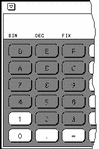## Display Notation

You can choose to display Calculator results in Fixed point, Engineering, or Scientific notation by using the Disp (Display) key menu shown in Figure 8-4. Press MENU on the Disp key to display the menu.

##### Figure 8-4 Display Type Pop-up Menu for Display Notation Mode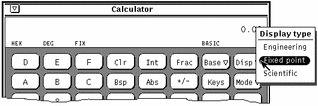The current display notation is displayed as the third item in the mode line. In Figure 8-4, the mode line shows FIX, indicating Fixed Point notation. When the current display notation is Engineering, ENG appears on the mode line. When the current notation is Scientific, SCI is displayed.

When the current notation is Fixed Point, the Calculator will revert to Scientific notation if a number is larger than the display will allow.

## Major Calculator Modes

There are four major Calculator modes that determine the available functions:

• Basic

• Financial

• Logical

• Scientific

You can choose these four modes using the Mode pop-up menu shown in Figure 8-5. Press MENU on the Mode key to display the menu. The default mode is Basic mode, which uses the standard keys on the Calculator window. The other three modes have extra buttons that appear in a pop-up window. For information on these modes, see "Financial Functions", "Logical Functions", and "Scientific Functions".

##### Figure 8-5 Mode Pop-up Menu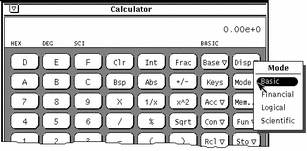##### Figure 8-6 The Mode Menu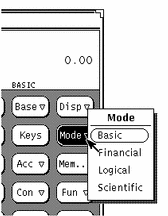The current major mode is displayed on the mode line underneath and slightly to the left of the number display. In Figure 8-5, BASIC is listed as the current mode.

## Number Manipulation Functions

The Calculator has 12 number manipulation functions, as shown in Figure 8-7.

##### Figure 8-7 Number Manipulation Functions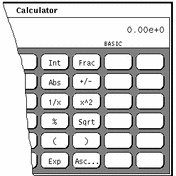• Int returns the integer portion of the currently displayed value.

• Frac returns the fractional portion of the currently displayed value.

• Abs returns the absolute value of the currently displayed value.

• +/- changes the arithmetic sign of the currently displayed value or of an exponent being entered with the Exp key.

• 1/x returns the value of 1 divided by the currently displayed value.

• x^2 returns the square of the currently displayed value.

• % calculates the percentage of the currently displayed value specified by the next value entered. For example, to find 75% of 40, enter 40, click SELECT on %, enter 75, then click SELECT on =. The Calculator returns the result, 30.

• Sqrt returns the square root of the currently displayed value.

• Parentheses give a calculation arithmetic precedence. All calculations within parentheses are calculated first. Parentheses can be nested. The result of an operation containing parentheses is not performed until the last parenthesis is matched.

• Exp starts exponential input. Any number entered after you choose Exp is taken to be the exponent portion of this number. The numbers entered before the Exp key are taken to be the mantissa. If no numerical input has occurred when the Exp key is entered, a mantissa of 1.0 is assumed. You can use the +/- key after entering the exponent to change its arithmetic sign.

• Asc displays the ASCII value of a character in the appropriate numeric base. Clicking on the Asc key displays a small pop-up window. Type the character that you want an ASCII equivalent for in the Character text field of the Get ASCII window, and then click SELECT on the ASCII button. The ASCII equivalent becomes the current value in the Calculator base window.